# Texas Go Math Grade 3 Unit 2 Answer Key Number and Operations: Multiplication and Division

Refer to our Texas Go Math Grade 3 Answer Key Pdf to score good marks in the exams. Test yourself by practicing the problems from Texas Go Math Grade 3 Unit 2 Answer Key Number and Operations: Multiplication and Division.

## Texas Go Math Grade 3 Unit 2 Answer Key Number and Operations: Multiplication and Division

Show What You Know

Check your understanding of important skills.

Count Equal Groups Complete.

Question 1.___ groups of ___
__ in all
Answer: 2 groups of 2. 4 in all.
Explanation:
Counted Equal Groups and dots to Complete

Question 2.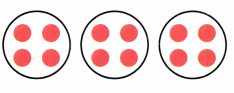__ groups of ___
__ in all
12 in all
Explanation:
Counted Equal Groups and dots to Complete

Question 3.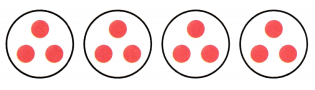__ groups of ___
__ in all
12 in all
Explanation:
Counted Equal Groups and dots to Complete

Question 4.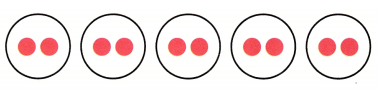__ groups of ___
__ in all
10 in all
Explanation:
Counted Equal Groups and dots to Complete

Skip Count by Twos and Fives Skip count. Write the missing numbers.

Question 5.
2, 4, 6, __, __, ___
Explanation:
Written the missing numbers to counted by Twos

Question 6.
5, 10, 15, __, __, __
Explanation:
Written the missing numbers to counted by Fives

Meaning of Division Complete.

Question 7.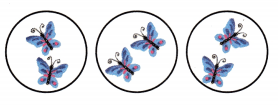___ groups
___ in each group
2 in each group
Explanation:
3 groups of 2 . So, total is 6
6 divided by 3 is 2. So 2 in each group.

Question 8.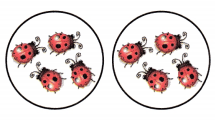__ groups
___ in each group
4 in each group
Explanation:
2 groups of 4 . So, total is 8
8 divided by 2 is 4. So 4 in each group.

Vocabulary Builder

Visualize It

Sort the words with a ✓ into the Venn diagram.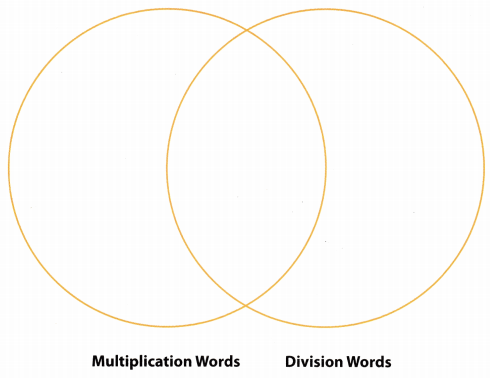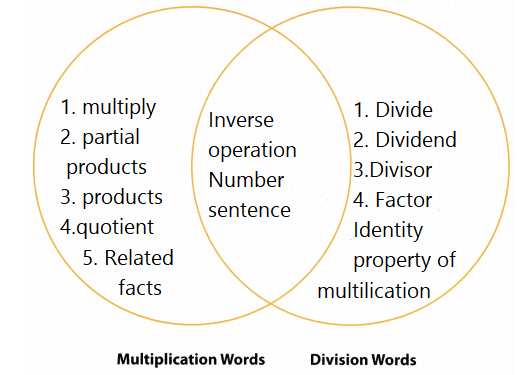Explanation:
Sorted the words

Understand Vocabulary

Complete the sentences by using the preview words.

Question 1.
An ____ is a set of objects arranged in rows and columns.
Explanation:
An array is an arrangement of a set of objects, pictures, or numbers usually arranged in rows and columns.

Question 2.
The ___ Property of Multiplication states that the product of any number and 1 is that number.
Explanation:
Identity property of multiplication: The product of 1 and any number is that number. For example, 7 × 1 = 7 7 times 1 = 7 7×1=77, times, 1, equals, 7.
Question 3.
The ___ Property of Multiplication states that when the order of the factors is changed, the product stays the same.Explanation:
Commutative property of multiplication states that the answer remains the same when multiplying numbers, even if the order of numbers are changed. Changing the order of multiplication doesn’t change the product.

Vocabulary

You will learn that using an array is a good way to show multiplication. Look at the arrays below. The product of 2 × 7 is the same as the product of 7 × 2.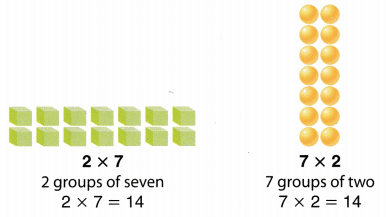Write two multiplication sentences for each array.

Question 1.Answer: 6 x3 =18 and 3 x 6 =18
Explanation:
6 groups of 3 is 18 and 3 groups of 6 is 18.

Question 2.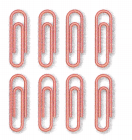Answer: 2 x4 =8 and 4 x 2 =8
Explanation:
2 groups of 4 is 8 and 4 groups of 2 is 8.

Question 3.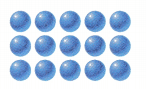Answer: 5  x 3 = 15 and 3 x 5 =15
Explanation:
5 groups of 3 is 15 and 3 groups of 5 is 15.

Writing Reread the example at the top of the page. How does this example show the Commutative Property of Multiplication?

Reading Check out this book in your library. Amanda Bean’s Amazing Dream: A Mathematical Story, by Cindy Neuschwander and Marilyn BurnsLet Me Count the Ways

Object of the Game Explore how to separate items into equal groups.

Materials

• 2 number cubes labeled 0-5
• 1-inch grid paper
• ones blocks

Number of Players 2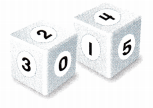How to Play

1. Player 1 tosses the number cubes. He or she then multiplies the 2 numbers tossed. The product is the number of ones blocks Player 1 will place in equal groups.
2. Player 1 places ones blocks to show the different ways to place them into equal groups.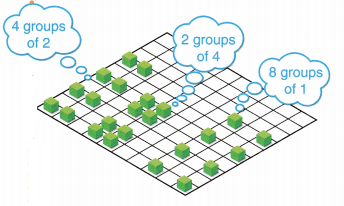3. Player 1 gets 1 point for each correct way the blocks are arranged in equal groups. No points are given for a single group of the product. Player 1 then removes all of his or her blocks.
4. Player 2 repeats Steps 1-3. The player who first earns a total of 20 points wins.Scroll to Top